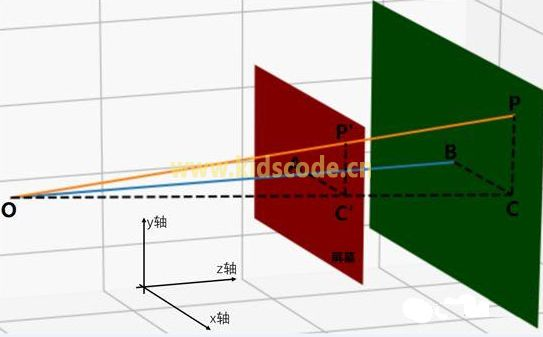OA /OB = AC’ / BC = P’C’ / PC

P点坐标x,y,z

O点坐标ox,oy,oz

OA = pz – oz

OB = z – oz

BC = x – ox

PC = y – oy

P’的x坐标 =ox + AC’

P’的x坐标=ox + [(pz – oz) * (x - ox)/(z - oz)]

P’的y坐标 =oy + P’C’

P’的y坐标=oy + [(pz - oz) * (y - oy)/(z - oz)]